• 为了能精确表示、计算浮点数，由于在运算的时候，float类型和double很容易丢失精度所以Java提供了BigDecimal类；该类提供了大量的构造器 用于创建BigDecimal对象，包括把所有的基本数值型变量转换成一个BigDecimal...
为了能精确表示、计算浮点数，由于在运算的时候，float类型和double很容易丢失精度所以Java提供了BigDecimal类；该类提供了大量的构造器 用于创建BigDecimal对象，包括把所有的基本数值型变量转换成一个BigDecimal对象，也包括利用数字字符串、数字字符数组来创建BigDecimal对象。由于在商业计算中,对数字精度要求较高,必须使用 BigInteger 类和 BigDecimal 类,它支持任何精度的定点数,可以用它来精确计算货币值。由于在运算的时候，float类型和double很容易丢失精度，所以一般不用来做计算货币。 BigDecimal类成员方法：
public BigDecimal add(BigDecimal augend)：加法public BigDecimal subtract(BigDecimal subtrahend)：减法public BigDecimal multiply(BigDecimal multiplicand)：乘法public BigDecimal divide(BigDecimal divisor)：除法public BigDecimal abs(BigDecimal abs)：绝对值 精度丢失，看程序结果：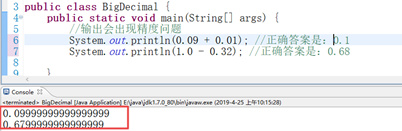1、public BigDecimal add(BigDecimal augend)：加法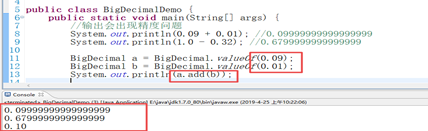用BigDecimal相加方法就不会出现精度丢失的问题出现； 2、 public BigDecimal subtract(BigDecimal subtrahend)：减法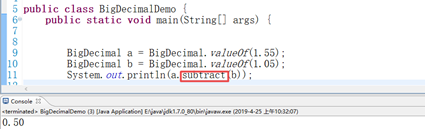3、 public BigDecimal multiply(BigDecimal multiplicand)：乘法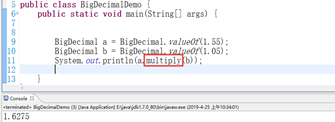4、 public BigDecimal divide(BigDecimal divisor)：除法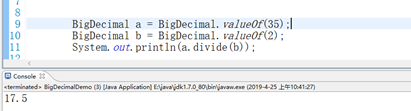5、 public BigDecimal abs(BigDecimal abs)：绝对值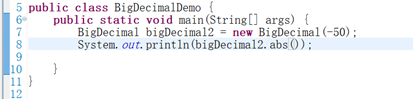结果：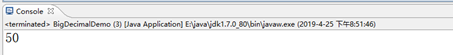常用的构造器： 1、 BigDecimal(char[] in)：将BigDecimal的字符数组表示形式转换为 BigDecimal，接受与 BigDecimal(String) 构造方法相同的字符序列。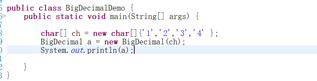结果：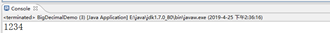2、 BigDecimal(double val)：将double转换为BigDecimal，后者是double的二进制浮点值准确的十进制表示形式。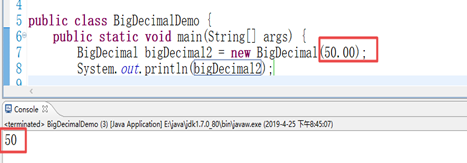3、 BigDecimal(int val)：将int转换为BigDecimal。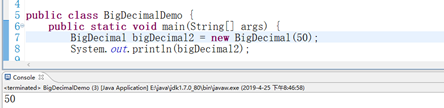展开全文• 介绍了JavaBigDecimal类...


目录
BigDecimal1. 概述2. 构造方法3. 常用方法

BigDecimal
使用基本类型做浮点数运算（即小数运算）会有精度问题，如果要解决这种精度问题，可以使用 BigDecimal；对于浮点运算，不要使用基本类型，而使用 BigDecimal 类；
public static void main(String[] args) {
System.out.println(0.09 + 0.01);//0.09999999999999999
System.out.println(1.0 - 0.32);//0.6799999999999999
System.out.println(1.015 * 100);//101.49999999999999
System.out.println(1.301 / 100);//0.013009999999999999
}

1. 概述
相关内容具体描述包java.math 使用时需要导包类声明public class BigDecimal extends Number implements Comparable描述BigDecimal类提供了算术，缩放操作，舍入，比较，散列和格式转换的操作。提供了更加精准的数据计算方式
注意 BigDecimal 不是继承 Math，而是继承自 Number；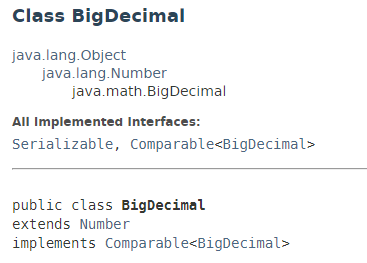2. 构造方法
推荐使用第二种方式，第一种存在精度问题；
构造方法名描述BigDecimal(double val)将 double 类型的数据封装为 BigDecimal 对象BigDecimal(String val)将 BigDecimal 的字符串表示形式转换为 BigDecimal

3. 常用方法
BigDecimal 类中使用最多的还是提供的进行四则运算的方法；
注意：对于 divide 方法来说，如果除不尽的话，就会出现 java.lang.ArithmeticException 异常，此时可以使用 divide 方法的另一个重载方法：BigDecimal divide(BigDecimal divisor, int scale, int roundingMode): divisor：除数； scale：精确的位数；roundingMode：取舍模式；
展开全文• 如果对数值结果精度要求很高，那么就可以使用BigDecimalBigDecimal可以精准的控制小数点后面的数字 1.实例BigDecimal 对象 通常会使用 String 和 int 构造方法实例出BigDecimal 对象，即 new BigDecimal(String...
如果对数值结果精度要求很高，那么就可以使用BigDecimal，BigDecimal可以精准的控制小数点后面的数字
1.  实例 BigDecimal 对象
通常会使用 String 和 int 构造方法实例出 BigDecimal 对象，即
new BigDecimal(String val); new BigDecimal(int val);   如参数为 double 类型，得先转换成 String 类型再入参
new BigDecimal(Double.toString(123.123));  2. BigDecimal 加减乘除
加减乘除都是用 BigDecimal 对象点（.）加减乘除方法入参 BigDecimal 对象，返回的也是 BigDecimal 对象
加：BigDecimal add = new BigDecimal("123.123").add(new BigDecimal("123.123"));  ---> 246.246 减：BigDecimal subtract = new BigDecimal("123.123").subtract(new BigDecimal("123.123"));  ---> 0.000 乘：BigDecimal multiply = new BigDecimal("123.123").multiply(new BigDecimal("123.123"));  ---> 15159.273129 除：BigDecimal divide = new BigDecimal("123.123").divide(new BigDecimal("123.123"));  ---> 1    注：其中除法一般不会这样直接除，在不能整除的情况下，这样运行程序是会报错的，所以一般除法会使用下面这个重载方法
new BigDecimal("10").divide("40",1,ROUND_HALF_UP);  ---> 0.3   正常计算 10/40 结果为 0.25， 在这里是计算 十除以四十，保留一位小数，四舍五入模式。所以得到的结果为 0.3
最常用的模式：
① ROUND_HALF_UP：四舍五入
② ROUND_HALF_DOWN：向下取舍（去掉保留小数位后面的小数）
3. Scale 属性操作
① 获取小数的位数：new BigDecimal("123.123").scale()  ---> 3
② setScale() 方法保留几位小数
- setScale(int NewScale)：（注：入参的数字只能大于等于小数的位数，括号中只能入参大于等于 3 的数）
例： new BigDecimal("123.123").setScale(3)  ---> 123.123
new BigDecimal("123.123").setScale(5)  ---> 123.12300
- setScale(int newScale, RoundingMode roundingMode)：
例：new BigDecimal("123.123").setScale(2,RoundingMode.HALF_UP)  ---> 123.12
new BigDecimal("123.125").setScale(2,RoundingMode.HALF_UP)  ---> 123.13
new BigDecimal("123.123").setScale(2,RoundingMode.HALF_DOWN)  ---> 123.12
new BigDecimal("123.125").setScale(2,RoundingMode.HALF_DOWN)  ---> 123.12
4. compareTo 比较大小
等于：new BigDecimal("123.123").compareTo(new BigDecimal("123.123"))==0  ---> true        new BigDecimal("123.123").compareTo(new BigDecimal("123.123"))  ---> 0
如果 compareTo 的结果为 0 的话，就证明两个对象相等（当左边不等于右边时，第一个表达式则为 false）
小于：new BigDecimal("123.122").compareTo(new BigDecimal("123.123")) < 0  ---> true        new BigDecimal("123.122").compareTo(new BigDecimal("123.123"))  ---> -1
如果 compareTo 的结果为 -1 的话，就证明左边小于右边（当左边大于等于右边时，第一个表达式则为 false）
大于：new BigDecimal("123.124").compareTo(new BigDecimal("123.123")) > 0  ---> true        new BigDecimal("123.124").compareTo(new BigDecimal("123.123"))  ---> 1
如果 compareTo 的结果为 1 的话，就证明左边大于右边（当左边小于等于右边时，第一个表达式则为 false） ———————————————— 版权声明：本文为CSDN博主「intomylife」的原创文章，遵循CC 4.0 BY-SA版权协议，转载请附上原文出处链接及本声明。 原文链接：https://blog.csdn.net/qq_41402200/article/details/86492437
展开全文• 所以我们一般使用BigDecimal来解决商业运算上丢失精度的问题的时候，声明BigDecimal对象的时候一定要使用它构造参数为String的类型的构造器。 同时这个原则Effective Java和MySQL 必知必会中也都有提及。float和...

前言

我们都知道浮点型变量在进行计算的时候会出现丢失精度的问题。如下一段代码：
System.out.println(0.05 + 0.01);
System.out.println(1.0 - 0.42);
System.out.println(4.015 * 100);
System.out.println(123.3 / 100);

输出：
0.060000000000000005
0.5800000000000001
401.49999999999994
1.2329999999999999

可以看到在Java中进行浮点数运算的时候，会出现丢失精度的问题。那么我们如果在进行商品价格计算的时候，就会出现问题。很有可能造成我们手中有0.06元，却无法购买一个0.05元和一个0.01元的商品。因为如上所示，他们两个的总和为0.060000000000000005。这无疑是一个很严重的问题，尤其是当电商网站的并发量上去的时候，出现的问题将是巨大的。可能会导致无法下单，或者对账出现问题。所以接下来我们就可以使用Java中的BigDecimal类来解决这类问题。
普及一下：
Java中float的精度为6-7位有效数字。double的精度为15-16位。

API

构造器：
  BigDecimal(int)       创建一个具有参数所指定整数值的对象。
BigDecimal(double)    创建一个具有参数所指定双精度值的对象。
BigDecimal(long)      创建一个具有参数所指定长整数值的对象。
BigDecimal(String)    创建一个具有参数所指定以字符串表示的数值的对象。

函数：
  add(BigDecimal)       BigDecimal对象中的值相加，然后返回这个对象。
subtract(BigDecimal)  BigDecimal对象中的值相减，然后返回这个对象。
multiply(BigDecimal)  BigDecimal对象中的值相乘，然后返回这个对象。
divide(BigDecimal)    BigDecimal对象中的值相除，然后返回这个对象。
toString()            将BigDecimal对象的数值转换成字符串。
doubleValue()         将BigDecimal对象中的值以双精度数返回。
floatValue()          将BigDecimal对象中的值以单精度数返回。
longValue()           将BigDecimal对象中的值以长整数返回。
intValue()            将BigDecimal对象中的值以整数返回。

由于一般的数值类型，例如double不能准确的表示16位以上的数字。

BigDecimal精度也丢失

我们在使用BigDecimal时，使用它的BigDecimal(String)构造器创建对象才有意义。其他的如BigDecimal b = new BigDecimal(1)这种，还是会发生精度丢失的问题。如下代码：
BigDecimal a = new BigDecimal(1.01);
BigDecimal b = new BigDecimal(1.02);
BigDecimal c = new BigDecimal("1.01");
BigDecimal d = new BigDecimal("1.02");

输出：
2.0300000000000000266453525910037569701671600341796875
2.03

可见论丢失精度BigDecimal显的更为过分。但是使用Bigdecimal的BigDecimal(String)构造器的变量在进行运算的时候却没有出现这种问题。究其原因计算机组成原理里面都有，它们的编码决定了这样的结果。long可以准确存储19位数字，而double只能准备存储16位数字。double由于有exp位，可以存16位以上的数字，但是需要以低位的不精确作为代价。如果需要高于19位数字的精确存储，则必须用BigInteger来保存，当然会牺牲一些性能。所以我们一般使用BigDecimal来解决商业运算上丢失精度的问题的时候，声明BigDecimal对象的时候一定要使用它构造参数为String的类型的构造器。
同时这个原则Effective Java和MySQL 必知必会中也都有提及。float和double只能用来做科学计算和工程计算。商业运算中我们要使用BigDecimal。
而且我们从源码的注释中官方也给出了说明，如下是BigDecimal类的double类型参数的构造器上的一部分注释说明：
     * The results of this constructor can be somewhat unpredictable.
* One might assume that writing {@codenew BigDecimal(0.1)} in
* Java creates a {@code BigDecimal} which is exactly equal to
* 0.1 (an unscaled value of 1, with a scale of 1), but it is
* actually equal to
* 0.1000000000000000055511151231257827021181583404541015625.
* This is because 0.1 cannot be represented exactly as a
* {@codedouble} (or, for that matter, as a binary fraction of
* any finite length).  Thus, the value that is being passed
* <i>in</i> to the constructor is not exactly equal to 0.1,
* appearances notwithstanding.
……
* When a {@codedouble} must be used as a source for a
* {@code BigDecimal}, note that this constructor provides an
* exact conversion; it does not give the same result as
* converting the {@codedouble} to a {@code String} using the
* {@link Double#toString(double)} method and then using the
* {@link #BigDecimal(String)} constructor.  To get that result,
* use the {@codestatic} {@link #valueOf(double)} method.
* </ol>
public BigDecimal(double val) {
this(val,MathContext.UNLIMITED);
}

第一段也说的很清楚它只能计算的无限接近这个数，但是无法精确到这个数。 第二段则说，如果要想准确计算这个值，那么需要把double类型的参数转化为String类型的。并且使用BigDecimal(String)这个构造方法进行构造。去获取结果。

正确运用BigDecimal

另外，BigDecimal所创建的是对象，我们不能使用传统的+、-、*、/等算术运算符直接对其对象进行数学运算，而必须调用其相对应的方法。方法中的参数也必须是BigDecimal的对象，由刚才我们所罗列的API也可看出。
在一般开发过程中，我们数据库中存储的数据都是float和double类型的。在进行拿来拿去运算的时候还需要不断的转化，这样十分的不方便。这里我写了一个工具类：
public class BigDecimalUtil {

private BigDecimalUtil() {

}

public static BigDecimal add(double v1, double v2) {// v1 + v2
BigDecimal b1 = new BigDecimal(Double.toString(v1));
BigDecimal b2 = new BigDecimal(Double.toString(v2));
}

public static BigDecimal sub(double v1, double v2) {
BigDecimal b1 = new BigDecimal(Double.toString(v1));
BigDecimal b2 = new BigDecimal(Double.toString(v2));
return b1.subtract(b2);
}

public static BigDecimal mul(double v1, double v2) {
BigDecimal b1 = new BigDecimal(Double.toString(v1));
BigDecimal b2 = new BigDecimal(Double.toString(v2));
return b1.multiply(b2);
}

public static BigDecimal div(double v1, double v2) {
BigDecimal b1 = new BigDecimal(Double.toString(v1));
BigDecimal b2 = new BigDecimal(Double.toString(v2));
// 2 = 保留小数点后两位   ROUND_HALF_UP = 四舍五入
return b1.divide(b2, 2, BigDecimal.ROUND_HALF_UP);// 应对除不尽的情况
}
}

展开全文• 当程序中涉及金钱存储变量的时候，可以通过BigDecimal进行操作，比int更加安全。 ps：微信支付时，只能用整形，价钱...//声明 BigDecimal moneys =new BigDecimal(money);//转换int int moneys1 = moneys.intValuestring 存储 微信
• Java的各种机试题中，经常会出现一些计算，这些计算的结果和运算数可能会超过内置数据类型的大小。这个时候就需要使用BigDecimal类，它可以进行大数的运算；1、BigDecimal类的初始化有两种方法初始化，一种是使用...
• ## JavaBigDecimal

千次阅读 2016-08-03 20:02:06
java.math.BigDecimal 类提供用于算术，刻度操作，舍入，比较，哈希算法和格式转换操作。 toString()方法提供BigDecimal的规范表示。它使用户可以完全控制舍入行为。 提供用于操作BigDecimal规模两种类型的操作：
• 如果在bean中属性是BigDecimal类型的，数据库中与其对应的字段想要一个空值，那么直接插入null就可以了。比如person.setBigDecimal(null);
• 一、大数值 ...浮点数运算 BigDecimal （1）BigInteger 代码示例： import java.math.*; public class BigInteger { public static void main(String[] args) { BigInteger b1 = new BigInteg...
• Double d1 = 1233.555;... * 这里有个坑，new BigDecimal的时候参数不要传Double类型，要转成String类型 * 参数Double类型：实际bd=1233.555000000000063664629124104976654052734375 * 参数String类型：实际b...
• 金额必须是完全精确的计算, 故不能使用double或者float, 而应该采用java.math.BigDecimal. 加减乘除： 两个BigDecimal值应该怎样进行加减乘除呢? +, -, *, / 这样写吗? 不! 加减乘除使用了英文的加减乘除, ...
• java使用BigDecimal 处理商业精度及高精度详解2016年11月15日 19:47:54阅读数：2645前言之前我是写过一篇类似笔记：java处理高精度的商业计算但是呢，写的太简单，关键还没有写到要点，所以重新写一篇。情形由于公司...
• 于是使用java中自带的BigDecimal类进行计算。 当然使用BigDecimal类与值类型的int、float等有所不同。 1、声明一个BigDecimal 　BigDecimal b1=new BigDecimal("10"); 这样就声明了一个引用b1指向值为10的...
• 技术交流QQ群【JAVA,C++,...Javajava.math包中提供的API类BigDecimal，用来对超过16位有效位的数进行精确的运算。双精度浮点型变量double可以处理16位有效数。在实际应用中，需要对更大或者更小的数进行运算和...
• 首先声明，在下新手。写博客只是为了记录和分享。 jint异常
• ## JavaBigDecimal详解

千次阅读 2018-11-06 10:10:56
Java BigDecimal详解 1.引言 　float和double类型的主要设计目标是为了科学计算和工程计算。他们执行二进制浮点运算，这是为了在广域数值范围上提供较为精确的快速近似计算而精心设计的。然而，它们没有提供完全...
• ## JavaBigDecimal篇

千次阅读 2018-07-12 14:04:02
BigDecimal 的家庭成员 1.在api中查看对象的关系，到需要的时候可以用父类来接受不同的值 2.看到Comparable接口，可以想到可以集合间排序比较 int compareTo（T o） 将此对象与指定的对象进行比较以获得顺序...
• Java中提供了大数字(超过16位有效位)的操作类,即 java.math.BinInteger 类和 java.math.BigDecimal 类,用于高精度计算. 其中 BigInteger 类是针对大整数的处理类,而 BigDecimal 类则是针对大小数的处理类. ...
• java.math包中提供了BigDecimal类，用来对超过16位有效位的数进行精确的运算。float和double只能用来做科学计算或工程计算，在商业计算中例如金额若需要精确的计算结果就要用到BigDecimal类。 一般的算术运算 ......

# java声明bigdecimaljava 订阅